# WO2011158893A1 - X-ray ct device and control method for same - Google Patents

## Info

Publication number
WO2011158893A1
WO2011158893A1 PCT/JP2011/063766 JP2011063766W WO2011158893A1 WO 2011158893 A1 WO2011158893 A1 WO 2011158893A1 JP 2011063766 W JP2011063766 W JP 2011063766W WO 2011158893 A1 WO2011158893 A1 WO 2011158893A1
Authority
WO
WIPO (PCT)
Prior art keywords
filter
means
convolution
projection data
value
Prior art date
Application number
PCT/JP2011/063766
Other languages
French (fr)
Japanese (ja)
Inventor

Original Assignee

Priority date (The priority date is an assumption and is not a legal conclusion. Google has not performed a legal analysis and makes no representation as to the accuracy of the date listed.)
Filing date
Publication date
Priority to JP2010-138576 priority Critical
Priority to JP2010138576 priority
Application filed by 株式会社 日立メディコ filed Critical 株式会社 日立メディコ
Publication of WO2011158893A1 publication Critical patent/WO2011158893A1/en

• 238000009499 grossing Methods 0 abstract claims description 67
• 238000001914 filtration Methods 0 abstract claims description 30
• 238000006243 chemical reaction Methods 0 claims description 78
• 238000000034 methods Methods 0 claims description 47
• 238000004364 calculation methods Methods 0 claims description 30
• 230000000875 corresponding Effects 0 claims description 14
• 230000002596 correlated Effects 0 claims description 3
• 230000004044 response Effects 0 claims 2
• 238000003384 imaging method Methods 0 description 41
• 230000037010 Beta Effects 0 description 20
• 230000001131 transforming Effects 0 description 19
• 238000003860 storage Methods 0 description 17
• 230000001276 controlling effects Effects 0 description 9
• 238000005315 distribution function Methods 0 description 8
• 239000011159 matrix materials Substances 0 description 7
• 230000001629 suppression Effects 0 description 6
• 210000000038 chest Anatomy 0 description 5
• 230000003287 optical Effects 0 description 5
• 230000002093 peripheral Effects 0 description 5
• 238000007781 pre-processing Methods 0 description 5
• 210000001015 Abdomen Anatomy 0 description 4
• 210000003128 Head Anatomy 0 description 3
• 230000003187 abdominal Effects 0 description 3
• 239000003570 air Substances 0 description 3
• 238000009826 distribution Methods 0 description 3
• 230000014509 gene expression Effects 0 description 3
• 210000003414 Extremities Anatomy 0 description 2
• 238000007792 addition Methods 0 description 2
• 239000002872 contrast medium Substances 0 description 2
• 230000001965 increased Effects 0 description 2
• 239000007924 injection Substances 0 description 2
• 238000002347 injection Methods 0 description 2
• 239000000203 mixtures Substances 0 description 2
• 239000007787 solids Substances 0 description 2
• 210000000481 Breast Anatomy 0 description 1
• 101700036312 CONA family Proteins 0 description 1
• 210000001624 Hip Anatomy 0 description 1
• 210000004279 Orbit Anatomy 0 description 1
• 230000001133 acceleration Effects 0 description 1
• 230000015572 biosynthetic process Effects 0 description 1
• 238000002591 computed tomography Methods 0 description 1
• 230000001419 dependent Effects 0 description 1
• 230000000694 effects Effects 0 description 1
• 239000011519 fill dirt Substances 0 description 1
• 230000001678 irradiating Effects 0 description 1
• 239000004973 liquid crystal related substances Substances 0 description 1
• 238000009740 moulding (composite fabrication) Methods 0 description 1
• 230000000399 orthopedic Effects 0 description 1
• 239000011295 pitch Substances 0 description 1
• 230000002829 reduced Effects 0 description 1
• 238000005070 sampling Methods 0 description 1
• 238000003786 synthesis Methods 0 description 1
• 238000001308 synthesis method Methods 0 description 1
• 230000002194 synthesizing Effects 0 description 1
• 238000007514 turning Methods 0 description 1

## Images

•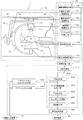•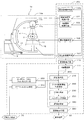•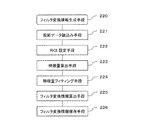•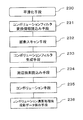•••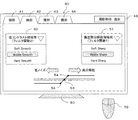•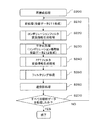••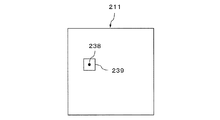•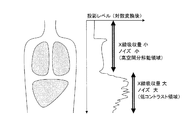•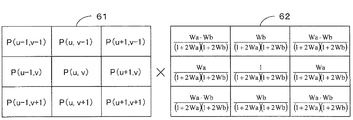•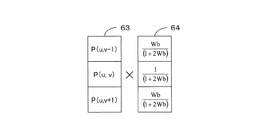•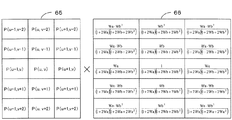•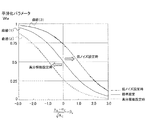•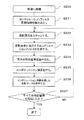••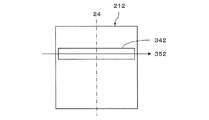••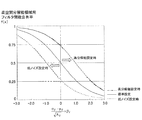•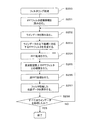## Classifications

• GPHYSICS
• G01MEASURING; TESTING
• G01NINVESTIGATING OR ANALYSING MATERIALS BY DETERMINING THEIR CHEMICAL OR PHYSICAL PROPERTIES
• G01N23/00Investigating or analysing materials by the use of wave or particle radiation not covered by groups G01N3/00 – G01N17/00, G01N21/00 or G01N22/00
• G01N23/02Investigating or analysing materials by the use of wave or particle radiation not covered by groups G01N3/00 – G01N17/00, G01N21/00 or G01N22/00 by transmitting the radiation through the material
• G01N23/04Investigating or analysing materials by the use of wave or particle radiation not covered by groups G01N3/00 – G01N17/00, G01N21/00 or G01N22/00 by transmitting the radiation through the material and forming images of the material
• G01N23/046Investigating or analysing materials by the use of wave or particle radiation not covered by groups G01N3/00 – G01N17/00, G01N21/00 or G01N22/00 by transmitting the radiation through the material and forming images of the material using tomography, e.g. computed tomography [CT]
• AHUMAN NECESSITIES
• A61MEDICAL OR VETERINARY SCIENCE; HYGIENE
• A61BDIAGNOSIS; SURGERY; IDENTIFICATION
• A61B6/02Devices for diagnosis sequentially in different planes; Stereoscopic radiation diagnosis
• A61B6/03Computerised tomographs
• A61B6/032Transmission computed tomography [CT]
• AHUMAN NECESSITIES
• A61MEDICAL OR VETERINARY SCIENCE; HYGIENE
• A61BDIAGNOSIS; SURGERY; IDENTIFICATION
• A61B6/52Devices using data or image processing specially adapted for radiation diagnosis
• A61B6/5205Devices using data or image processing specially adapted for radiation diagnosis involving processing of raw data to produce diagnostic data
• GPHYSICS
• G06COMPUTING; CALCULATING; COUNTING
• G06TIMAGE DATA PROCESSING OR GENERATION, IN GENERAL
• G06T11/002D [Two Dimensional] image generation
• G06T11/003Reconstruction from projections, e.g. tomography
• G06T11/006Inverse problem, transformation from projection-space into object-space, e.g. transform methods, back-projection, algebraic methods
• AHUMAN NECESSITIES
• A61MEDICAL OR VETERINARY SCIENCE; HYGIENE
• A61BDIAGNOSIS; SURGERY; IDENTIFICATION
• A61B6/52Devices using data or image processing specially adapted for radiation diagnosis
• A61B6/5258Devices using data or image processing specially adapted for radiation diagnosis involving detection or reduction of artifacts or noise
• GPHYSICS
• G06COMPUTING; CALCULATING; COUNTING
• G06TIMAGE DATA PROCESSING OR GENERATION, IN GENERAL
• G06T2211/00Image generation
• G06T2211/40Computed tomography
• G06T2211/421Filtered back projection [FBP]
• YGENERAL TAGGING OF NEW TECHNOLOGICAL DEVELOPMENTS; GENERAL TAGGING OF CROSS-SECTIONAL TECHNOLOGIES SPANNING OVER SEVERAL SECTIONS OF THE IPC; TECHNICAL SUBJECTS COVERED BY FORMER USPC CROSS-REFERENCE ART COLLECTIONS [XRACs] AND DIGESTS
• Y10TECHNICAL SUBJECTS COVERED BY FORMER USPC
• Y10STECHNICAL SUBJECTS COVERED BY FORMER USPC CROSS-REFERENCE ART COLLECTIONS [XRACs] AND DIGESTS
• Y10S378/00X-ray or gamma ray systems or devices
• Y10S378/901Computer tomography program or processor

## Abstract

Disclosed are an X-ray CT device and a control method for same, for generating X-ray CT images with optimum image quality for each portion and each region of an examinee, when scanning the examinee across a plurality of portions using a plane detector. The X-ray CT device is characterized by comprising: an X-ray source (11) which generates X rays; an X-ray detector (12); a rotation means (13) that rotates the X-ray source (11) and the X-ray detector (12), whilst maintaining the mutually facing arrangement of same; a smoothing means (230) and a filtering means (250) that generate a convolution filter based on the characteristics amount in projection data output from the X-ray detector (12) and superimpose the convolution filter on the projection data; a reconfiguration means (200) that makes reconfiguration calculations for the projection data on which the convolution filter is superimposed and generates an X-ray CT image of the examinee; and an image display means (280) that displays the image generated by the reconfiguration means (200).

## Description

X-ray CT apparatus and control method thereof

The present invention relates to an X-ray CT apparatus, and in particular, an X-ray CT image that generates an optimal X-ray CT image for each part and each region of a subject when a subject that spans multiple parts is scanned with a surface detector. The present invention relates to a line CT apparatus and a control method thereof.

Generally, the output value of the X-ray detector becomes smaller as the body thickness is scanned, and the error becomes larger. For this reason, the noise of the reconstructed X-ray CT image becomes larger as the body thickness increases. On the other hand, a conventional X-ray CT apparatus normally generates an X-ray CT image of a subject using one reconstruction filter for one CT scan. For this reason, even if an optimal X-ray CT image can be provided for a certain part of the subject, the image quality may not be optimal for other regions.

In such a case, a plurality of reconstructed images in which a plurality of reconstructed filters such as a smoothing filter and a sharpening filter are superimposed are calculated, and the CT is calculated at each point for each reconstructed image. An X-ray CT apparatus is known that outputs an X-ray CT image obtained by combining a plurality of reconstructed images by setting and adding an addition coefficient corresponding to a value (Patent Document 1).

JP 2006-34785 A

However, the above-described X-ray CT apparatus has a problem that it takes a computation time because the reconstructed image is calculated for a plurality of reconstruction filters. In particular, when a flat panel detector is used for the X-ray detector, since the amount of noise in the imaging data is large, whether the smoothing filter or the sharpening filter should be used depends on the X-ray absorption at each reconstruction point. There is a problem that a good reconstructed CT image cannot be obtained unless the image quality (noise) of the reconstructed image is determined in addition to the coefficient (so-called CT value).

In view of the above problems, an object of the present invention is to provide an X-ray CT apparatus that suppresses an increase in calculation time and generates an X-ray CT image having an optimum image quality for each part.

The present invention generates an image processing filter that continuously changes based on the value of projection data, and performs image reconstruction calculation, thereby suppressing an increase in calculation time and optimal X-ray CT for each part. Realize an X-ray CT system that generates images.

More specifically, the X-ray CT apparatus according to the present invention includes an X-ray source that generates X-rays, and the X-ray that is disposed to face the X-ray source and transmits the subject to detect the X-ray. An X-ray detector that outputs the projection data, a rotating unit that rotates the X-ray source and the X-ray detector while facing each other, and image processing according to a feature value of a pixel value included in the projection data Filter generating means for generating a filter, reconstruction means for performing an reconstruction operation on the projection data using the generated image processing filter, and generating an X-ray CT image of the subject, and the X-ray And an image display means for displaying a CT image.

According to the present invention, in cone beam CT imaging in which a subject extending over a plurality of parts is scanned with a surface detector, an increase in calculation time is suppressed, and an X-ray CT image having an optimum image quality for each part and each region of the subject. Can be provided.

For example, in cone-beam CT scanning from the chest to the abdomen, a high spatial resolution X-ray CT image is obtained in the chest region where the amount of X-ray absorption is small, and the abdomen with a large X-ray absorption amount and a small X-ray detector output value An X-ray CT apparatus capable of generating an X-ray CT image excellent in low contrast resolution in the region can be realized.

1 is a schematic configuration diagram showing a cone beam X-ray CT apparatus (C-arm system) 1 to which the present invention is applied. 1 is a schematic configuration diagram showing a C-arm type cone beam X-ray CT apparatus 1a mounted on a mobile X-ray apparatus to which the present invention is applied. 5 is a block diagram showing components of filter conversion information generation means 220. FIG. 3 is a block diagram showing components of smoothing means 230. FIG. 3 is a block diagram showing components of filtering means 250. FIG. 5 is a schematic diagram showing an example of a convolution filter setting screen 30. FIG. 4 is a schematic diagram showing an example of an input filter setting screen 40. FIG. 6 is a flowchart showing a flow of reconstruction processing (S200) executed by the reconstruction means 200. It is a flowchart which shows the flow of a convolution filter conversion information generation process (S220). It is explanatory drawing which shows the feature-value calculation area | region and the coordinate point on projection data. It is a conceptual diagram which shows the relationship between the value of projection data, and an imaging | photography site | part. It is explanatory drawing explaining a convolution calculation when a convolution filter size is 3x3. It is explanatory drawing explaining a convolution calculation in case a convolution filter size is 1x3. It is explanatory drawing explaining a convolution calculation when a convolution filter size is 3x5. 6 is an explanatory diagram showing a curve indicated by a function of standard deviation σc of projection data 211 and a smoothing parameter Wa. FIG. It is a flowchart which shows the flow of a smoothing process (S230). It is a flowchart which shows the flow of a process of FFT filter conversion information generation processing (S240). It is explanatory drawing which shows a filtering process. 5 is an explanatory diagram showing an example of an FFT filter function input by an FFT filter input means 340 and an FFT filter function generated by an FFT filter generation means 253. FIG. It is explanatory drawing which shows the curve which the function of standard deviation (sigma) and the filter function content rate for high spatial resolution area | region shows. It is a flowchart which shows the flow of a process of a filtering process (S250).

Hereinafter, embodiments of the X-ray CT apparatus according to the present invention will be described in detail with reference to the accompanying drawings. In all the drawings for explaining the embodiments of the present invention, those having the same function are denoted by the same reference numerals, and repeated explanation thereof is omitted.

<Outline configuration>
First, a schematic configuration of a cone beam X-ray CT apparatus to which the present invention is applied will be described with reference to FIGS. 1-1 and 1-2. FIG. 1-1 is a schematic configuration diagram showing a cone beam X-ray CT apparatus (C-arm system) 1 to which the present invention is applied. FIG. 1-2 is a schematic configuration diagram showing a C-arm type cone beam X-ray CT apparatus 1a mounted on a mobile X-ray apparatus to which the present invention is applied.

A cone beam X-ray CT apparatus 1 shown in FIG. 1-1 irradiates a subject 2 with X-rays, and captures an X-ray transmission image 111 of the subject 2 and each configuration of the imaging unit 10 And a control calculation unit 20 that controls elements and reconstructs a three-dimensional CT image of the subject 2 based on the X-ray transmission image 111. In addition, a display device 80 that displays an image and an information input device 70 that includes a mouse, a keyboard, a trackball, or the like for inputting the position and parameters of the image displayed on the display device 80 are provided.

The C-arm type cone-beam X-ray CT apparatus 1a mounted on the mobile X-ray apparatus shown in Fig. 1-2 controls each component of the imaging unit 10a and the imaging unit 10a, and reproduces a 3D CT image. Or a control operation unit 20a configured. The cone beam X-ray CT apparatus 1a is equipped with wheels 5 so that it can move between the examination room and the operating room.

By the way, in Fig. 1-1, there is a rotation center axis 4 in a direction parallel to the paper surface, and the X-ray source 11 and the two-dimensional X-ray detector 12 rotate around the rotation center axis 4. On the other hand, Fig. 1-2 is drawn such that the rotation center axis 4 exists in a direction perpendicular to the paper surface, and the X-ray source 11 and the two-dimensional X-ray detector 12 rotate in a plane parallel to the paper surface. However, even if the cone beam X-ray CT apparatus 1 in FIG. 1-1 slides and rotates in a plane parallel to the paper surface, the cone beam X-ray CT apparatus 1a mounted on the movable X-ray apparatus in FIG. May turn.

Hereinafter, the components shown in Fig. 1-1 will be mainly explained, and if necessary, the components shown in Fig. 1-2 will be explained.

(Shooting unit 10)
The imaging unit 10 includes a bed 17, an X-ray source 11 that irradiates the subject 2 lying on the bed 17 with X-rays, and an X-ray that is installed opposite to the X-ray source 11 and passes through the subject 2 A two-dimensional X-ray detector 12 that outputs an X-ray transmission image 111 by detecting the X-ray, a C-type arm 13 that mechanically connects the X-ray source 11 and the two-dimensional X-ray detector 12, and the C-type arm C-type arm holding body 14 for holding 13, a ceiling support 15 for attaching the C-type arm holding body 14 to the ceiling, and supporting the ceiling support 15 so as to be movable in the two-dimensional direction of front, rear, left and right in the illustrated state. A ceiling rail 16 and an injector 18 for injecting a contrast medium into the subject 2.

The X-ray source 11 includes an X-ray tube 11t that generates X-rays, and a collimator 11c that controls the direction of X-ray irradiation from the X-ray tube 11t to be a cone, a quadrangular pyramid, or a multi-sided pyramid.

For the two-dimensional X-ray detector 12, for example, a flat panel detector (hereinafter referred to as “FPD”) using a TFT element is used. Further, as another example of the two-dimensional X-ray detector 12, an X-ray image intensifier that converts an X-ray transmission image into a visible light image, an optical lens that forms an image of the X-ray image intensifier, and You may use the two-dimensional X-ray detector comprised from the combination of the CCD television camera etc. which image | photograph the visible light image of the X-ray image intensifier imaged by the optical lens. The imaging field of view of the two-dimensional X-ray detector 12 may be any shape such as a circle or a rectangle.

The C-arm 13 rotates around the rotation center axis 4 at every predetermined projection angle when the subject 2 is imaged. As a result, while the X-ray source 11 and the two-dimensional X-ray detector 12 are arranged to face each other, a circular orbit on substantially the same plane is rotated and moved to perform X-ray imaging. For this rotational movement, there are imaging geometry parameters that are used for the image reconstruction calculation. The imaging geometric parameters include a rotation orbit plane (midplane) 3 that is a plane including a circular orbit drawn by the X-ray source 11 and a rotation center axis 4 as the C-arm 13 rotates and moves.

(Control calculation unit 20)
The control calculation unit 20 includes an imaging unit control unit 100 that controls the imaging unit 10, an image collection unit 110 that collects and stores the X-ray transmission image 111 output from the imaging unit 10, and the collected X-ray transmission image 111. Reconstructing means 200 for reconstructing a 3D CT image based on the above, an image display means 280 for displaying the 3D CT image generated by the reconstructing means 200, and for the reconstructing means 200 to generate a convolution filter The convolution filter input means 320 for inputting the generation conditions to be used, and the FFT filter for inputting the generation conditions used by the reconstruction means 200 to generate a Fourier transform (hereinafter referred to as FFT) filter. Input means 340. The convolution filter is a coefficient that is superimposed on the pixel value and its surrounding pixel values when performing image processing such as smoothing and sharpening using convolution calculation in the image space. An FFT filter is a coefficient that is subjected to FFT conversion for each line (one-dimensional) in a two-dimensional image space and is superimposed for each frequency in line data generated by the FFT conversion. Details of the generation conditions used to generate the convolution filter and the generation conditions used to generate the FFT filter will be described later with reference to FIGS.

(Shooting unit control means 100)
The imaging unit control means 100 controls the position of the C-arm 13 on the ceiling rail 16 and the imaging system rotation control means 101 that controls the rotational movement of the C-arm 13 around the rotation center axis 4 to control the C An imaging system position control means 102 that two-dimensionally controls the position of the mold arm 13 with respect to the subject 2, an X-ray irradiation control means 103 that controls ON, OFF, etc. of the tube current flowing through the X-ray tube 11t, and an injector 18 Injector control means 104 for controlling the injection amount and injection timing of contrast medium injected into the subject 2, a bed control means 105 for adjusting the position of the subject 2 by controlling the position of the bed 17, and a two-dimensional And detection system control means 107 for controlling imaging of the X-ray transmission image 111 by the X-ray detector 12. As described above, the rotation direction of the C-arm 13 has the rotation center axis 4 in a direction parallel to the paper surface, and the X-ray source 11 and the two-dimensional X-ray detector 12 rotate around the rotation center axis 4. (Fig. 1-1) so that the rotation center axis 4 exists in a direction perpendicular to the paper surface, and the X-ray source 11 and the two-dimensional X-ray detector 12 slide and rotate in a plane parallel to the paper surface. (FIGS. 1-2) or both of them may be provided.

(Reconstruction means 200)
The reconstruction unit 200 includes a preprocessing unit 210, a filter conversion information generation unit 220, a smoothing unit 230, a filtering unit 250, and a back projection unit 260.

The pre-processing means 210 converts the X-ray transmission image 111 collected by the image collecting means 110 into an X-ray absorption coefficient distribution image (hereinafter referred to as “projection data 211”). In the present embodiment, first, natural logarithmic conversion calculation is performed on each pixel data of an X-ray transmission image of air that has been captured in advance without the subject 2 and the bed 17 being placed in the field of view. Next, a natural logarithmic conversion operation is performed on each pixel data of an X-ray transmission image taken with the subject 2 placed on the bed 17. Then, by subtracting the X-ray transmission image of the subject 2 (and the bed 17) subjected to the natural logarithmic conversion calculation from the X-ray transmission image of the air subjected to the natural logarithmic conversion calculation, the projection data Get 211.

Next, based on FIG. 2, filter conversion information generation means included in the cone-beam X-ray CT apparatus (C-arm type) 1 in FIG. 1-1 and the C-arm type cone-beam X-ray CT apparatus 1a in FIG. The 220 components will be described. FIG. 2 is a block diagram showing components of the filter conversion information generation means 220 in the present invention.

The filter conversion information generation unit 220 is a unit that characterizes the present invention, and is used by the smoothing unit 230 and the filtering unit 250 to generate a convolution filter and an FFT filter on a frequency space as an image processing filter. Generate filter conversion parameters. The filter conversion information generation unit 220 includes a projection data reading unit 221, an ROI setting unit 222, a feature amount calculation unit 223, a feature amount fitting unit 224, a filter conversion information calculation unit 225, and a filter conversion information storage unit 226. . Each of these components is configured by software that realizes the function of each component, and hardware including an arithmetic / control device, an input / output device, and a storage device that executes the software. Cooperates with each other to realize the function of each component.

Projection data reading means 221 reads the projection data 211 generated by the preprocessing means 210. The ROI setting means 222 sets the calculation area of the projection data 211. The feature amount calculating unit 223 calculates the feature amount (average value, standard deviation, etc.) of the pixel values in the vicinity of each point of the projection data 211 in the calculation area set by the ROI setting unit 222. The feature amount fitting unit 224 fits the feature amount of each point of the projection data 211 calculated by the feature amount calculation unit 223 as a function of the coordinate value of the projection data. The filter conversion information calculation unit 225 converts the fitting result of the feature amount fitting unit 224 into a convolution filter parameter. The filter conversion information storage unit 226 stores the filter conversion parameter as a function of the coordinate value of the projection data. It is not necessary to calculate the feature amount at all points on the image of the projection data 211. It is sufficient to set the calculation area and calculate the feature amount for the points thinned out vertically and horizontally. The filter conversion parameters for all points of the projection data 211 can be obtained by the mapping unit 224 and the filter conversion information calculation unit 225.

Next, based on FIG. 3, the smoothing means 230 included in the cone beam X-ray CT apparatus (C arm method) 1 in FIG. 1-1 and the C arm type cone beam X-ray CT apparatus 1a in FIG. The components will be described. FIG. 3 is a block diagram showing components of the smoothing means 230. As shown in FIG.

The smoothing unit 230 uses the convolution filter generation conditions input by the convolution filter input unit 320 and the filter conversion parameters generated by the filter conversion information generation unit 220 and stored in the filter conversion information storage unit 226. A convolution filter is generated for each point of the projection data 211, and a two-dimensional convolution operation is performed on the projection data 211. As shown in FIG. 3, the smoothing unit 230 includes a convolution filter conversion information reading unit 231, a pixel scanning unit 232, a convolution filter generation unit 233, a peripheral pixel reading unit 234, a convolution unit 235, and a convolution calculation process. The post projection data storage means 236 is used. Each of these components is configured by software that realizes the function of each component, and hardware including an arithmetic / control device, an input / output device, and a storage device that executes the software. Cooperates with each other to realize the function of each component.

The convolution filter conversion information reading means 231 reads the filter conversion parameter generated by the filter conversion information generation means 220. The pixel scanning unit 232 scans the coordinate values of the projection data 211 (coordinates and pixel values of the coordinates), and the convolution filter generation unit 233 calculates each of the projection data from the convolution filter generation conditions and the filter conversion parameters. A convolution filter corresponding to the point is generated. The pixel scanning unit 232 scans the projection data 211 and reads the coordinate values (coordinates and pixel values of the coordinates) of each point of the projection data.

The peripheral pixel reading means 234 is based on a coordinate value scanned by the pixel scanning means 232 (hereinafter referred to as a “peripheral pixel”) near a point that is a convolution filter generation target (hereinafter referred to as a “projection pixel”). ) Projection data value (pixel value). The convolution means 235 generates a convolution generated by the convolution filter generation means 233 for the projection pixel value (pixel value), the projection pixel value read by the peripheral pixel reading means 234, and the peripheral pixel value. A convolution operation is performed by applying a filter. The projection data storage means 236 after the convolution calculation process stores the convolution calculation result.

Next, based on FIG. 4 and FIG. 15, “filtering included in“ cone beam X-ray CT apparatus (C-arm type) 1 ”in FIG. 1-1 and C-arm type cone beam X-ray CT apparatus 1a in FIG. The components of the means 250 will be described: Fig. 4 is a block diagram showing the components of the filtering means 250. Fig. 15 is an explanatory diagram showing the filtering process.

The filtering unit 250 uses the FFT filter generation conditions input by the FFT filter input unit 340 and the filter conversion parameters generated by the filter conversion information generation unit 220 to perform projection data (hereinafter referred to as “convolution”). An FFT filter corresponding to each horizontal line 212 (referred to as “projection data after arithmetic processing”) is generated and subjected to FFT filter processing. As shown in FIG. 4, the filtering means 250 includes an FFT filter conversion information reading means 251, a line data reading means 252, an FFT filter generating means 253, an FFT means 254, an FFT filter integrating means 255, an inverse FFT means 256, and a filter process. The post projection data storage means 257 is configured. Each of these components is configured by software that realizes the function of each component, and hardware including an arithmetic / control device, an input / output device, and a storage device that executes the software. Cooperates with each other to realize the function of each component.

The FFT filter conversion information reading means 251 reads the filter conversion parameters generated by the filter conversion information generation means 220 and stored in the filter conversion information storage means 226. The line data reading means 252 reads horizontal line data to be filtered at a time from the projection data 212, for example, horizontal line data 352 in FIG. The FFT filter generation means 253 generates an FFT filter for each line from the FFT filter generation conditions and the filter conversion parameters. The FFT means 254 converts the horizontal line data 352 into frequency data, and the FFT filter integration means 255 integrates the FFT filter generated by the FFT filter generation means 253 on the frequency data. The inverse FFT unit 256 returns the frequency data to the real space data, and the filter processing result is stored by the post-filtering projection data storage unit 257.

The back projection means 260 performs back projection calculation of the filtered projection data and generates a 3D CT image of the subject 2.

(Convolution filter input means 320)
The convolution filter input means 320 sets conditions for generating a two-dimensional convolution filter generated by the convolution filter generation means 233. Hereinafter, an example of the GUI used by the convolution filter input unit 320 will be described with reference to FIG. FIG. 5 is a schematic diagram showing an example of the convolution filter setting screen 30. As shown in FIG.

Tabs 41 to 44 in FIG. 5 are imaging region selection tabs, and convolution filter generation conditions can be set separately for various imaging regions such as the head, chest, abdomen, and waist. 5 represents a case where the chest condition setting tab 42 is selected. A button 45 is an imaging region addition button, and conditions for other imaging regions such as a neck and limbs can be added. The list box 31 is a list box for selecting a convolution filter size in the horizontal direction, and a value of “1”, “3”, or “5” can be selected.

The list box 32 is a list box for selecting a convolution filter size in the vertical direction, and a value of “1”, “3”, or “5” can be selected. However, when the convolution filter size “1” is selected in the list boxes 31 and 32, it means that the horizontal or vertical convolution calculation is not performed (OFF setting).

Points 33 and 35 in FIG. 5 are horizontal or vertical convolution filter function threshold setting points that can be dragged to the left or right to change the horizontal filter function threshold μa or the vertical filter function threshold μb. ing. Here, the “filter function threshold value” is a value that defines how much smoothing processing is performed (in other words, how much sharpening processing is not performed). When the user desires a relatively low-noise image, it is necessary to execute the smoothing process relatively large (in other words, execute the sharpening process relatively small). In this case, the filter function threshold is set to a relatively small value. On the other hand, when the user desires a relatively high resolution image, it is necessary to execute the smoothing process relatively small (in other words, execute the sharpening process relatively large). In this case, the filter function threshold is set to a relatively large value.

Points 34 and 36 are horizontal or vertical convolution filter function change amount setting points. By changing the slope of the filter function threshold boundary line, the horizontal filter function change amount βa or the vertical filter is changed. The function change amount βb can be changed. The “filter function change amount” here defines the change amount between the filter function applied when the smoothing process is relatively increased and the filter function region used when the smoothing process is relatively reduced. The value to be The relationship between the magnitude of the filter function threshold and the magnitude of the smoothing process, and the relation between the magnitude of the filter function change amount and the magnitude of the smoothing process will be further described in “Fermi distribution function parameters” described later. Details of the process in which the convolution filter generation unit 233 generates a convolution filter using the filter function threshold values μa and μb and the filter function change amounts βa and βb will be described later.

(FFT filter input means 340)
The FFT filter input unit 340 sets the generation conditions for the FFT filter generated by the FFT filter generation unit 253. Hereinafter, an example of the GUI used by the FFT filter input unit 340 will be described with reference to FIG. FIG. 6 is a schematic diagram illustrating an example of the FFT filter setting screen 40. Similarly to FIG. 5, tabs 41 to 44 are imaging region selection tabs, and a button 45 is an imaging region addition button. FIG. 6 shows a case where the chest condition setting tab 42 is selected.
The list box 51 is a list box for selecting a filter function for a high spatial resolution region, and the list box 52 is a list box for selecting a filter function for a low contrast region, and selects a generation condition used by the FFT filter generation means 253. Point 53 is the FFT filter function threshold setting point, and point 54 is the FFT filter function change setting point, and the FFT filter function changes by dragging left and right to change the FFT filter function threshold μ F or the threshold boundary slope. The amount β F can be changed. The “FFT filter function threshold μ F ” here is a value that defines the level of smoothing processing using the FFT filter. The “FFT filter function change amount β F ” is a value that defines the rate of change between the two filter functions (filter function 1 and filter function 2 in FIG. 6) used in the FFT filter processing. Details of processing in which the FFT filter generation unit 253 generates an FFT filter using the filter function threshold μ F , the filter function change amount β F , and the selected high spatial resolution region filter function and low contrast region filter function Will be described later.

The specification examples of the cone beam X-ray CT apparatuses 1 and 1a are as follows. The distance between the X-ray source 11 and the rotation center axis 4 is 800 mm, the distance between the rotation center axis 4 and the X-ray incident surface of the two-dimensional X-ray detector 12 (FPD) is 400 mm, and the X-ray incidence surface is 400 mm × 300 mm. The size is a rectangle, the number of TFT elements is 2048 × 1536, and the element interval is 0.2 mm. When X-rays enter the FPD, the light is first converted into light by a light emitter such as CsI on the X-ray incident surface, and the optical signal is converted into electric charge by a photodiode. The accumulated charge is converted into a digital signal by a TFT element at a constant frame rate and read out. In the rotational imaging mode, a 2 × 2 TFT element is binned, and the X-ray transmission image 111 is read out at an image size of 1024 × 768, a pixel pitch of 0.4 mm, and 30 frames per second. The imaging system rotation control means 101 passes the two-dimensional X-ray detector 12 from the direction of the left hand (−100 degrees) of the subject 2 to the ceiling direction (0 degrees) and to the right hand direction (+100 degrees) of the subject 2 To move. Thereby, an X-ray transmission image 111 of the subject 2 over a projection angle of 200 degrees is taken. The rotational speed of the C-arm 13 is 40 degrees per second, for example, and the scan time is 5 seconds.

<Overview of operation>
Next, an outline of operations in imaging by the cone beam X-ray CT apparatus 1 will be described.

In the cone beam X-ray CT apparatus 1, first, the imaging system rotation control means 101 starts to turn the C-arm 13 around the rotation center axis 4. After the rotation acceleration period, the X-ray irradiation control means 103 emits X-rays from the X-ray tube 11t, and the detection system control means 107 starts imaging by the two-dimensional X-ray detector 12. X-rays irradiated from the X-ray tube 11t pass through the subject 2 and are taken into the two-dimensional X-ray detector 12. The signal from the two-dimensional X-ray detector 12 undergoes A / D conversion, and is then recorded in the image acquisition means 110 as an X-ray transmission image 111 composed of a digital signal. The standard scanning mode of the two-dimensional X-ray detector FPD is 30 frames per second, the projection angle interval in rotational imaging is 1.33 degrees, and 150 X-ray transmission images 111 are acquired in 5 seconds. When the rotation imaging at 200 degrees is completed, the X-ray irradiation control unit 103 ends the X-ray irradiation of the X-ray tube 11t, and the imaging system rotation control unit 101 stops the rotation after a rotation deceleration period.

Also, for example, as a two-dimensional X-ray detector 12, when using a combination of an X-ray image intensifier, an optical lens and a CCD TV camera, the X-ray image intensifier has a diameter of 300 mm, a CCD TV camera The standard scan mode is 60 frames per second and 512 scanning lines, or 30 frames per second and 1024 scanning lines. The CCD TV camera uses a visible light image of an X-ray image intensifier imaged by an optical lens. Take a picture. An X-ray transmission image taken by the CCD TV camera is converted into a video signal, A / D converted, and collected as 512 × 512 or 1024 × 1024 digital image by the image collecting means 110.

The reconstruction unit 200 reads the X-ray transmission image 111 from the image acquisition unit 110 during the above-described rotation imaging operation or immediately after the end of the rotation imaging, performs a reconstruction operation based on the X-ray transmission image 111, and performs the calculation. A 3D CT image of specimen 2 is generated. The image display means 280 displays a three-dimensional CT image on a display device 80 such as a CRT device or a liquid crystal display device. The image display means 280 is also used to display the X-ray transmission image 111 recorded in the image collection means 110. The reconstruction unit 200 generates a reconstructed image of the subject by reconstructing the X-ray transmission image 111 collected by the image collection unit 110, and the image display unit 280 displays the reconstructed image on the display device 80. indicate.

<Reconfiguration process>
Next, the flow of the reconstruction process (S200) executed by the reconstruction unit 200 will be described with reference to FIG. FIG. 7 is a flowchart showing the flow of the reconstruction process (S200) executed by the reconstruction unit 200. Hereinafter, description will be given along the order of steps in FIG.

(Step S210)
The preprocessing unit 210 performs a natural logarithmic conversion operation on the X-ray transmission image 111 of the subject 2 and air collected by the image collection unit 110, and converts it into projection data 211 (S210).

(Step S220)
The filter conversion information generation unit 220 calculates the feature amount (for example, the average value Ac and standard deviation σc of the pixel values of the pixels in the vicinity of each point) of the projection data 211, and generates a convolution filter in step S230. A convolution filter conversion parameter is generated for this purpose (S220).

(Step S230)
The smoothing means 230 uses the convolution filter conversion parameters generated in step S220 and the convolution filter generation conditions set in advance by the user using the convolution filter input means 320, to generate each point of the projection data 211. A convolution filter is generated for each point, and a two-dimensional convolution operation is performed for each point (S230).

(Step S240)
The filter conversion information generation means 220 calculates the feature values (average value A F , standard deviation σ F ) of the pixel values of each horizontal line data of the projection data 212 subjected to the convolution calculation processing, and performs the FFT filter in step S250. An FFT filter conversion parameter for generation is generated (S240).

(Step S250)
The filtering unit 250 uses the FFT filter conversion parameters generated in step S240 and the FFT filter generation conditions set in advance by the user using the FFT filter input unit 340, and the projection data 212 subjected to the convolution calculation process. An FFT filter corresponding to each horizontal line is generated and subjected to FFT filter processing (S250).

(Step S260)
The back projection means 260 performs a back projection operation using the projection data after the FFT filter processing in step S250 (S260).

(Step S270)
It is determined whether or not the processing from step S210 to step S260 has been executed for all projection data. If all the projection data has not been processed (NO), the process returns to step S210, and the processes from step S210 to step S260 are executed for the next projection data. If YES, the reconstruction process (S200) is finished, and a 3D CT image of the subject 2 is output (S270).

Hereinafter, the details of the processes of steps S220, S230, S240, and S250 that characterize the present invention will be described with reference to FIGS. 8, 9, 10, and 12. FIG. FIG. 8 is a flowchart showing the flow of the convolution filter conversion information generation process (S220), FIG. 9 is an explanatory diagram showing the feature amount calculation area and the coordinate points on the projection data, and FIG. FIG. 12 is an explanatory diagram illustrating a curve indicated by a function of the standard deviation σc of the projection data 211 and the smoothing parameter Wa.

First, the convolution filter conversion information generation process will be described along each step of FIG.

(Step S221)
The projection data reading means 221 reads the projection data 211 generated in step S210 (S221).

(Step S222)
As shown in FIG. 9, the ROI setting means 222 sets the size of a rectangular or square ROI (calculation area 239) for calculating the feature amount of the projection data 211. The ROI size is, for example, 15 × 15 to 25 × 25 pixels centered on the coordinate point 238 (S222).

(Step S223)
The feature amount calculating means 223 scans the coordinate points on the projection data 211, and calculates the average value Ac and the standard deviation σc of the pixel values in the ROI area (calculation area 239) designated in step S222, thereby projecting. The feature amount of each point in the data 211 is obtained. At this time, in order to prevent the calculated value of the standard deviation from being affected by the data greatly deviating from the average value, the horizontal axis represents the average value, maximum value, and minimum value of the pixel values within that ROI once for each ROI. Create a histogram. Then, the standard deviation value may be obtained using only the data within the frequency distribution of, for example, ± 1/10 from the average value of the pixel values in the ROI (S223). In the present embodiment, the feature amount is obtained for each point of the projection data 211. However, the feature amount may be obtained only for the points that are thinned out appropriately without obtaining the feature amount for all the points. In this case, the feature value of the pixel value obtained from the calculation area including the point may be used as the feature value of the thinned projection data 211.

FIG. 10 conceptually shows changes in the value of the projection data in imaging of a region extending from the chest to the abdomen. Since the amount of X-ray absorption is small in the chest region, the projection data after logarithmic transformation has a small average value and a small error (standard deviation). On the other hand, in the abdominal region, the projection data after logarithmic transformation has a large average value and a small output value from the X-ray detector, and therefore has a large error.

The present invention generates a high spatial resolution X-ray CT image at an imaging region where the average value of the projection data is small and the standard deviation is small, and a smoothing filter at an imaging region where the error of the projection data is large. To achieve an X-ray CT system that can generate X-ray CT images with low contrast and excellent low-contrast resolution.

(Step S224)
The feature amount fitting unit 224 fits the feature amount of each point of the projection data 211 calculated in step S223 as a function of the coordinate value of the projection data (that is, the horizontal and vertical positions of each point of the projection data 211). Information (coordinate values) is associated with the feature value of the pixel value at that position) (S224). Note that steps S223 and S224 may be omitted. In this case, for example, based on the imaging region and imaging conditions, the user inputs the predicted value of the feature value (or feature value range) of the pixel value, and using the feature value of the input pixel value, the following Filter conversion information may be calculated.

(Step S225)
The filter conversion information calculation means 225 converts the fitting result of step S224 into a convolution filter parameter, that is, generates a convolution filter parameter using the feature value of the pixel value included in the fitting result (S225). . Details of the parameters of the convolution filter and the processing thereof will be described below with reference to FIG. FIG. 12 is an explanatory diagram showing a curve indicated by a function of the standard deviation σc of the projection data 211 and the parameter Wa.

Generally, the convolution filter is standardized so that the sum is 1. Parameters that can be changed under the condition that the total number of convolution filters is 1, for example, the parameter Wa in the horizontal direction (the u direction in FIGS. 11-1 to 11-3 described later) and the vertical direction (described later in FIG. 11-1). The parameter Wb in the v direction in FIGS. 11-1 to 11-3 can be introduced. The horizontal parameter Wa and the vertical parameter Wb are real values exceeding -0.5, and 1.0 is usually the maximum value.

The convolution operation when the parameter Wa or the parameter Wb takes a negative value is an operation that takes a difference from an adjacent pixel, and in this case, acts as a sharpening filter. In the description of the present embodiment, it is assumed that the parameter Wa and the parameter Wb take positive values (0.0 to 1.0), the convolution operation functions as a smoothing filter, and the sharpening process is performed later on the FFT filter. It will be described below as being performed in the calculation. Therefore, in the following description of the smoothing process, the parameter Wa or the parameter Wb is described as the smoothing parameter Wa or the smoothing parameter Wb.

Hereinafter, from the feature amount (ROI average value Ac, standard deviation σc) of the projection data 211 calculated by the feature amount calculation unit 223 in step S223, the filter conversion information calculation unit 225 in step S225 sets the parameters Wa and Wb. An example of the function formula to be defined will be described. As described above, the smoothing parameters Wa and Wb are assumed to take values of 0.0 to 1.0. On the other hand, the characteristic amount (ROI average value Ac, standard deviation σc) of projection data is typically 0.02 / mm (water X-ray absorption coefficient) × 200 mm (body thickness) = 4.0, where Ac is σc takes a value of about 0.2, but assumes any real value. As an example of a function whose input is an arbitrary real value and whose output is 0 to 1, the following “Fermi distribution function” f (x) is introduced.

Wa = f (x) or the function f (y)

Wb = f (y) can be set. In the expressions (1) and (2), μa and μb are horizontal and vertical filter function threshold values input by the convolution filter input means 320, and βa and βb are filter function change amounts. From the following, βa and βb should be standard values of about 10. The “Fermi distribution function” in Equation (1) and Equation (2) has the property of f (x) + f (−x) = 1, and the configuration exemplified in FIGS. 11-1 to 11-3 described later. In the calculation for calculating the volume filter, 1−Wa = f (−x) or 1−Wb = f (−y) may be used.

Curve (1) in FIG. 12 (drawn with a solid line in FIG. 12) indicates “Fermi distribution function” f (x), and when x = 0, that is, standard deviation σc = filter function threshold μa, f (0) = 0. 5. f (x) has a value of about 0.73 when x = −1.0, 0.5 when x = 0, and about 0.27 when x = + 1.0. The smoothing parameter Wa determined by the function f (x) has a large standard deviation σc (that is, noise amount) of the projection data when the ROI average value Ac, the filter function threshold μa, and the filter function change amount βa of the projection data do not change. As x decreases, the smoothing parameter Wa increases. That is, in general, in the “Fermi distribution function” f (x), when the filter function threshold μ = standard deviation σ, the smoothing parameter Wa = 0.5, and when μ <σ, Wa> 0.5, μ> σ When Wa <0.5, the smoothing process is relatively large when Wa> 0.5, and the smoothing process is relatively small when Wa <0.5. In addition, when the filter function change amount β (β> 0) is relatively increased under the condition of μ> σ, x becomes relatively large, so that the smoothing parameter W becomes relatively small and stronger high resolution. Processing is set. On the other hand, when the filter function change amount β (β> 0) is relatively increased under the condition of μ <σ, since the x becomes relatively small, the smoothing parameter W becomes relatively large and stronger low noise. Processing is set.

Curve (2) in FIG. 12 (depicted by a dotted line in FIG. 12) shows the filter function threshold μa when the average value Ac and the filter function change amount βa are standard values Ac = 4.0 and βa = 10. Shows the change of f (x) when ± is 0.2. When Ac = 4.0 and βa = 10, if the filter function threshold μa is +0.2, x in Equation (1) becomes 1.0 larger, and the smoothing parameter Wa becomes smaller for the same standard deviation σc. Thus, high resolution processing is set.

Conversely, when the filter function threshold μa is −0.2, x in Expression (1) is reduced by 1.0, the smoothing parameter Wa is increased, and low noise processing is set. As a result, compared to the standard curve (1), low noise is set, which corresponds to the curve (3) in FIG. 12 (drawn with a dotted line in FIG. 12).

The above is a description of the case in which the horizontal smoothing parameter Wa is calculated from the filter function threshold μa, the filter function change amount βa, the ROI average value Ac, and the standard deviation σc. The smoothing parameter Wb in the vertical direction is calculated by an expression in which 12 is replaced by a → b.

As described above, it is desirable from the viewpoint of the spatial resolution of the CT image to suppress the horizontal smoothing parameter Wa to a small value and increase the vertical smoothing parameter Wb. In FIG. 5, the horizontal filter function threshold μa indicated by the point 33 is set with a relatively large value, and the vertical filter function threshold μb indicated by the point 35 is set with a relatively small value. Is shown.

The processing of this step is performed for each point of the projection data 211, and information in which the coordinate value of each point is associated with the smoothing parameters Wa and Wb is generated.

(Step S226)
The filter conversion information storage unit 226 stores the filter conversion information (information using the convolution filter conversion parameter as a function of the coordinate value of the projection data) obtained in step S225 (S226).

Next, details of the smoothing process (S230) will be described with reference to FIGS. 11-1, 11-2, 11-3, and 13. FIG. Fig. 11-1 illustrates the convolution operation when the convolution filter size is 3x3, and Fig. 11-2 illustrates the convolution operation when the convolution filter size is 1x3. FIG. 11-3 is an explanatory diagram for explaining the convolution calculation when the convolution filter size is 3 × 5, and FIG. 13 is a flowchart showing the flow of the smoothing process (S230).
Hereinafter, description will be made along the order of steps in FIG.

(Step S231)
The convolution filter conversion information reading means 231 includes the convolution filter conversion information generated by the convolution filter conversion information generation process (S220) (specifically, the smoothing data stored as a function of the coordinate value of the projection data in step S226). Are read (S231).

(Step S232)
The pixel scanning unit 232 scans coordinate values (corresponding to pixel coordinates and pixel values) of each point (hereinafter referred to as a projection pixel) of the projection data 211 (S232).

(Step S233)
The convolution filter generation means 233 reads out the smoothing parameters Wa and Wb corresponding to the coordinate values of each projection pixel using the convolution filter conversion information, and generates a convolution filter corresponding to each projection pixel using them. (S233).

Here, with reference to FIG. 11-1 to FIG. 11-3 and FIG. 12, the process of the convolution calculation and the convolution filter generation means 233 in the present embodiment will be described with an example. Note that the convolution calculation and the convolution filter generation method are not limited to the following, and can be appropriately changed without departing from the technical idea of the present invention.

Fig. 11-1 shows that the horizontal and vertical convolution filter sizes are both "3", and Fig. 11-2 shows that the horizontal convolution filter size is "1" (i.e., the horizontal convolution filter size). If the vertical convolution filter size is "3", Fig. 11-3 shows that the horizontal convolution filter size is "3" and the vertical convolution filter size is "5". It is a figure which shows typically the convolution calculation performed in a case. Matrixes 61, 63, and 65 in FIG. 11-1 represent pixel values P (u, v) on which the convolution calculation is performed and pixel values of neighboring points. Matrixes 62, 64 and 66 represent convolution filters. 11-1 corresponds to the pixel value P (u, v) before the convolution calculation based on the following equation (3) and FIG. 11-2 based on the following equation (4). A pixel value P ′ (u, v) after the convolution calculation is calculated.

11-3, the same as the expression (3) in FIG. 11-1 and the expression (4) in FIG. 11-2, the pixel value of the matrix 65 is the same as the position of the pixel value in the matrix 65. The pixel value P ′ (u, v) after the convolution calculation is calculated by multiplying the weight in the matrix 66 at the position.

The convolution filters described in the matrices 62, 64 and 66 are the convolution filters using the smoothing parameter Wa and the smoothing parameter Wa generated by the filter conversion information generation means 220, and the sum thereof is 1. Has been standardized.

The spatial resolution of the reconstructed CT image greatly depends on the resolution of the projection data 211 in the horizontal direction (u direction). Therefore, the smoothing parameter Wa in the horizontal direction (u direction) may be suppressed to 0 or a small value, and the smoothing parameter Wb in the vertical direction (v direction) may be increased. Further, as illustrated in FIGS. 11-1 to 11-3, the size of the convolution filter may be 3 × 3 as a standard, and may be 1 × 3 or 3 × 5. In the present embodiment, since the convolution filter size in the horizontal direction is 3 and the convolution filter size in the vertical direction is 3 in the list boxes 31 and 32 of the convolution filter setting screen 30 in FIG. A three-size convolution filter is generated for each point of the projection data 211.

(Step S234)
The peripheral pixel reading means 234 reads a pixel value of a projection pixel (also referred to as a peripheral pixel) located around the projection pixel to be subjected to the convolution calculation (S234). Projection pixels located in the periphery are used for the following convolution calculations.

(Step S235)
The convolution means 235 performs a convolution operation using the convolution filter generated in step S233 and the pixel values of the projection pixel and the peripheral pixels read in steps S232 and S234 (S235). For example, when a 3 × 3 filter size is set on the convolution filter setting screen in FIG. 5, the convolution means 235 performs a convolution operation based on Expression (3).

(Step S236)
The projection data storage means 236 after the convolution calculation process stores the convolution calculation result of step S236 (S236).

(Step S237)
It is determined whether or not the processing from step S232 to step S236 has been executed for all projection pixels. If all the projection pixels have not been processed (NO), the process returns to step S232, and the processes from step S232 to step S236 are executed for the next projection pixel. In the case of YES, the smoothing process (S230) is finished, and the process proceeds to the FFT filter conversion information generation process (S240).

Next, details of the FFT filter conversion information generation processing (S240) will be described based on FIGS. 14 and 15. FIG. FIG. 14 is a flowchart showing the flow of the FFT filter conversion information generation process (S240), and FIG. 15 is an explanatory diagram showing the filtering process. Note that the vertical line 24 in FIG. 15 is a line indicating the projection of the rotation center axis 4 on the two-dimensional X-ray detector 12, and the filtering process is performed in the horizontal direction along the rotation direction (direction orthogonal to the rotation center axis 4). This is performed for the line data 352. Hereinafter, description will be given along the order of steps in FIG.

(Step S241)
The projection data reading means 221 reads the projection data 212 after the convolution calculation process generated in step S230 (S241).

(Step S242)
The ROI setting means 222 sets the size of the horizontally long rectangular ROI (calculation area 342) as shown in FIG. 15 (S242).

(Step S243)
The feature amount calculation means 223 scans the rectangular ROI (calculation region 342) in the vertical direction (or also referred to as the vertical direction) on the projection data 212 after the convolution calculation process, and the feature amount (for example, the pixel value of the pixel in the ROI) Average value A F and standard deviation σ F ). At this time, as in the case of the calculation in step S223, in order to prevent the calculated value of the standard deviation from being affected by the data greatly deviating from the average value, once the average value and maximum value of the pixel values in the ROI A histogram with the minimum value as the horizontal axis may be created, and the standard deviation value may be obtained using only the data within the frequency distribution of, for example, ± 1/10 from the average value of the pixel values in the ROI. (S243).

(Step S244)
The feature amount fitting unit 224 fits the feature amount calculated in step S243 as a function of the coordinate value related to the vertical direction of the projection data (that is, the positional information of the projection data in the vertical direction, the feature amount at the position, (S244).

(Step S245)
The filter conversion information calculation means 225 converts the fitting result of step S244 into the FFT filter parameters. Details of the parameters of the FFT filter and its processing will be described below (S245).

Here, with reference to FIG. 16 and FIG. 17, the FFT filter function and its synthesis method in the present embodiment will be described with an example. FIG. 16 is an explanatory diagram showing an example of the FFT filter function input by the FFT filter input means 340 and the FFT filter function generated by the FFT filter generation means 253, and FIG. 17 shows the standard deviation σ and the filter for the high spatial resolution region It is explanatory drawing which shows the curve which a function shows with a function content rate. Note that the FFT filter function and the synthesis method thereof are not limited to the following, and can be changed as appropriate without departing from the technical idea of the present invention.

FIG. 16 shows an example of the FFT filter function input by the FFT filter input means 340 and the FFT filter function generated by the FFT filter generation means 253. The top curve (a) and bottom curve (b) in FIG. 16 are the FFT filter function for the high spatial resolution region and the FFT filter for the low contrast region, which are input by the FFT filter input means 340. It is an example of a function. The middle three curves (depicted by dotted lines) are examples of FFT filter functions generated from the linear sum of two input FFT filter functions by the FFT filter generation means 253 in accordance with the feature amount of the projection data. The vertical axis represents the integrated intensity in the frequency space executed by the FFT filter integrating means 255, the horizontal axis represents the frequency, and the unit is [1 / Pixel].

The input image of the present embodiment is a 1024 × 768 image collected by 2 × 2 binning, which is a well-known fact in the FFT calculation, but is twice the width 1024 of the input image so as not to cause so-called aliasing. Assume that FFT filter operation is performed at 2048 points. At this time, the absolute value of the upper limit frequency (referred to as “Nyquist frequency”) is 1024 from the sample interval, and the frequency takes a value of −1024 to +1024. The FFT filter function is set to take the same value at positive and negative frequencies having the same absolute value, and FIG. 16 shows the FFT filter function having only a positive frequency. In the example of FIG. 16, the Shepp-Logan function is used as the FFT filter function for the high spatial resolution region, and the sine function having a half cycle is used as the FFT filter function for the low contrast region. The generated FFT filter function is expressed as a linear sum of the two functions. In the high spatial resolution region where the average value of the projection data is small and the standard deviation is small, the FFT filter having a large value up to a high frequency has a large X-ray absorption coefficient. In a low contrast region where the error of the projection data is large, an FFT filter having a small high frequency value and a large smoothing degree is generated. In all the FFT filter functions, the origin inclinations (differential coefficients) are set to be equal so that the average values of the reconstructed CT images are equal.

Next, using FIG. 17, the FFT filter generation unit 253 calculates a high value from the feature amount (ROI average value A F , standard deviation σ F ) of the projection data 212 after the convolution calculation process calculated in step S243. An example of a function formula that determines the content of the FFT filter function for the high spatial resolution region is the processing to generate the FFT filter using the linear sum of the FFT filter function for the spatial resolution region and the FFT filter function for the low contrast region. List and explain. The content ratio of the two input FFT filter functions (for the high spatial resolution region and for the low contrast region) in the generated FFT filter is 1 in total. On the other hand, as described above, the characteristic amount (ROI average value A F , standard deviation σ F ) of the projection data is typically A F is 0.02 / mm (water X-ray absorption coefficient) × 200mm (body thickness) = 4.0, σ F takes a value of about 0.2, but it is assumed to take any real value. As an example of a function whose input is an arbitrary real value and whose output is 0 to 1, the same “Fermi distribution function” f (x) as in equation (1)

(The definition expression of the variable x is different from the expression (1)), and f (x) can be set as the content rate of the FFT filter function for the high spatial resolution region. μ F is an FFT filter function threshold value input by the FFT filter input means 340, and β F is a filter function change amount. As described above, β F is set to a value of about 10 as a standard. The “Fermi distribution function” has a property of f (x) + f (−x) = 1, and f (−x) can be set as the content rate of the FFT filter function for the low contrast region.

Hereinafter, the discussion is the same as in FIG. 12 (however, the definition equation of variable x is different from equation (1)), the solid line in FIG. 17 is the `` Fermi distribution function '' f (x), and the FFT filter for the high spatial resolution region When the ROI average value A F of the projection data, the filter function threshold μ F , and the filter function change amount β F do not change, the content rate f (x) of the function is the standard deviation σ F (that is, the noise amount) of the projection data. The larger the value, the larger x and the smaller f (x). The dotted lines in FIG. 17 indicate that the filter function threshold μ F is ± 0.2 when the average value A F and the filter function change amount β F are standard values A F = 4.0 and β F = 10, respectively. Shows the change in f (x). When A F = 4.0 and β F = 10, if the filter function threshold μ is +0.2, x in Equation (5) is reduced by 1.0, and for the same standard deviation σ F, for high spatial resolution region The content rate f (x) of the FFT filter function is increased, and high resolution processing is set. Conversely, when the filter function threshold μ F is −0.2, x in Expression (5) is increased by 1.0, the content rate f (x) of the FFT filter function for the high spatial resolution region is reduced, and low noise processing is performed. Is set. f (x) is about 0.73 when x = -1.0, 0.5 when x = 0, and about 0.27 when x = +1.0. The FFT filter function is the three curves illustrated in FIG. This processing is performed for each line data, and is composed of data corresponding to the position information (coordinate values) in the vertical direction (vertical direction) of the line data and the content rate f (x) of the FFT filter function for the high spatial resolution region. Generate FFT filter conversion information. In the FFT filter conversion information, the content rate of the FFT filter function may be defined as a function of coordinate values in the vertical direction of the projection data.

(Step S246)
The filter conversion information storage unit 226 stores the FFT filter conversion information obtained in step S245 (S246).

Next, details of the filtering process (S250) will be described with reference to FIG. 15 and FIG. FIG. 15 is an explanatory diagram showing the filtering process, and FIG. 18 is a flowchart showing the process flow of the filtering process (S250). In the following, description will be given along the order of steps in FIG.

(Step S251)
The FFT filter conversion information reading means 251 is the FFT filter conversion information generated by the FFT filter conversion information generation process (S240) (specifically, the high-frequency data stored as a function of the coordinate values in the vertical direction of the projection data in step S246). The spatial resolution region FFT filter function content) is read (S251).

(Step S252)
The line data reading means 252 reads horizontal line data to be filtered at a time, for example, horizontal line data 352 in FIG. 15, from the projection data 212 after the convolution calculation process (S252).

(Step S253)
For each line data, the FFT filter generation means 253 corresponds to the vertical coordinates of the line data using the vertical coordinate values of the line data and the FFT filter conversion information read in step S251.
An FFT filter is generated (S253). The FFT filter generation means 253 calculates the FFT filter function content for the high spatial resolution region corresponding to the vertical coordinate values of the line data based on the FFT filter conversion information. The FFT filter function for the high spatial resolution region set and input by the user on the GUI screen of FIG. 6 is included according to the calculated content rate, and the FFT filter for the low contrast region set and input by the user on the GUI screen of FIG. An FFT filter including the function according to (1-the above content rate) is generated (S253).

(Step S254)
The FFT means 254 converts the horizontal line data 352 into frequency data (S254).

(Step S255)
The FFT filter integration means 255 integrates the FFT filter generated in step S253 on the frequency data (S255).

(Step S256)
The inverse FFT means 256 converts the frequency data into post-filter projection data in real space (S256).

(Step S257)
The post-filtering projection data storage unit 257 stores the post-filtering projection data generated in step S256 (S257).

(Step S258)
It is determined whether or not the processing from step S252 to step S257 has been executed for all the horizontal line data. If all the line data has not been processed (NO), the process returns to step S252, and the processes from step S252 to step S257 are executed for the next line data. In the case of YES, the filtering process (S250) is finished and the process proceeds to the back projection process (S260).

The embodiment of the present invention has been described above. However, the configuration described above is merely an example, and for example, the smoothing unit 230 and the convolution filter conversion information generation process (S220) are omitted, and the embodiment relates to the present embodiment. Perform only FFT filter conversion information generation processing and FFT filter processing using the same, or simplify the arithmetic processing by performing only the smoothing means 230 and the convolution filter conversion information generation processing (S220). Changes can be made as appropriate without departing from the technical idea. The former case can be realized by replacing the projection data 212 after the convolution calculation process in the above-described embodiment with the projection data 211. Further, although the average value and standard deviation of the pixel values in the calculation area are used as the feature value of the pixel value, the feature value is not limited to the average value or the standard deviation, for example, the mode value instead of the average value The median may be used instead of the standard deviation. Then, by using values in place of these for the average value and the standard deviation value of Equations (1) to (5), the same operational effects as in the above embodiment can be obtained.

According to the present invention, by generating a convolution filter for each point of the projection data, a convolution filter that continuously changes along the vertical and horizontal directions of the projection data is generated. An FFT filter that continuously changes along the vertical direction of projection data or projection data after convolution calculation processing by generating an FFT filter corresponding to each horizontal line data of projection data or projection data after convolution calculation processing Is generated. Then, by applying these continuously changing filters and performing convolution calculation processing and filtering processing, a sharpening filter is applied to the imaging region where the average value of the projection data is small and the standard deviation is small, and high spatial resolution is achieved. In addition to generating X-ray CT images, we provide an X-ray CT system that can generate X-ray CT images with excellent contrast and low-contrast resolution by applying a smoothing filter to areas with large projection data errors. It can be expected to improve the diagnostic performance of contrast imaging of the head, abdomen, etc., and orthopedic imaging of the teeth, jaws, lumbar vertebrae, and limbs.

In addition, since the convolution filter and the FFT filter whose parameters change continuously for the projection data value and the coordinate value are applied, the high spatial resolution CT image reconstruction area and the low contrast CT image reconstruction area It is possible to generate an X-ray CT image that does not produce an unnatural boundary line between them.

1 Cone beam X-ray CT device, 1a C-arm type cone beam X-ray CT device mounted on mobile X-ray device, 2 subject, 3 rotating orbital plane (midplane), 4 rotating central axis, 5 wheels, 10 Imaging unit, 10a Imaging unit of C-arm type cone-beam X-ray CT apparatus 1a mounted on mobile X-ray device, 11 X-ray source, 11t X-ray tube, 11c collimator, 12 2D X-ray detector, 13C Type arm, 14 C type arm holder, 15 ceiling support, 16 ceiling rail, 17 bed, 18 injector, 20 control arithmetic unit, 20a Control of C arm type cone beam X-ray CT1a mounted on mobile X-ray device Arithmetic unit, 24 Projection of the rotation center axis 4 to the two-dimensional X-ray detector 12, 30 Convolution filter setting screen, 31 Horizontal convolution filter size selection list box, 32 Vertical convolution filter size selection list box , 33 Horizontal direction convolution filter function threshold setting point, 34 Horizontal direction convolution filter function change setting point, 35 Vertical convolution filter function threshold setting point, 36 Vertical convolution filter function change setting point, 40 FFT filter Setting screen, 41 Head condition setting tab, 42 Chest condition setting tab, 43 Abdominal condition setting tab, 44 Lumbar condition setting tab, 45 Imaging region addition button, 51 Filter function selection list box for high spatial resolution area, 52 Low contrast area Filter function selection list box, 53 FFT filter function threshold setting point, 54 FFT filter function change amount setting point, 61 Pixel for which convolution calculation is performed when the convolution filter size is 3 × 3 and its neighboring points Value, 62 3 x 3 Convolution filter, 63 Convolution filter size is 1 × 3 Pixels to be subjected to convolution operation and pixel values of neighboring points, 64 1 × 3 convolution filter, 65 Convolution filter size is 3 × 5 Pixels to be subjected to convolution calculations and their neighboring pixel values, 66 3 × 5 convolution filter, 70 information input device, 80 display device, 100 imaging unit control means, 100a Mobile X-ray device Imaging unit control means, 101 imaging system rotation control means, 102 imaging system position control means, 103 X-ray irradiation control means, 104 injector control means, 105 bed control means, 107 of the C-arm type cone beam X-ray CT apparatus 1a Detection system control means, 110 image collection means, 111 X-ray transmission image, 200 reconstruction means, 210 preprocessing means, 211 projection data, 212 convolution Projection post-processing calculation data, 220 filter conversion information generation means, 221 projection data reading means, 222 ROI setting means, 223 feature quantity calculation means, 224 feature quantity fitting means, 225 filter conversion information calculation means, 226 filter conversion information storage Means, 230 smoothing means, 231 convolution filter conversion information reading means, 232 pixel scanning means, 233 convolution filter generation means, 234 peripheral pixel reading means, 235 convolution means, 236 projection data storage means after convolution calculation processing, 238 Coordinate points on projection data, 239 feature value calculation ROI, 250 filtering means, 251 FFT filter conversion information reading means, 252 line data reading means, 253 FFT filter generating means, 254 FFT means, 255 FFT filter integrating means, 256 inverse FFT means, 257 projection after filtering Data storage means, 260 back projection means, 280 image display means, 320 convolution filter input means, 340 FFT filter input means, 342 feature amount calculation ROI, 352 horizontal line data

## Claims (10)

1. An X-ray source that generates X-rays;
An X-ray detector that is arranged opposite to the X-ray source, detects the X-ray transmitted through the subject, and outputs projection data of the subject;
Rotating means for rotating the X-ray source and the X-ray detector while facing each other,
Filter generation means for generating an image processing filter that changes in accordance with a feature value of a pixel value included in the projection data;
Reconstruction means for performing reconstruction operation on the projection data using the generated image processing filter, and generating an X-ray CT image of the subject;
Image display means for displaying the X-ray CT image;
An X-ray CT apparatus comprising:
2. The filter generation means generates a convolution filter for each point of the projection data as the image processing filter corresponding to the feature value of the pixel value of the point,
The reconstruction means comprises convolution means for superimposing the convolution filter for each point.
2. The X-ray CT apparatus according to claim 1, wherein
3. The X-ray CT apparatus has a first input means for inputting a generation condition of the convolution filter,
First filter conversion information generating means for generating first filter conversion information including a parameter that changes in accordance with the feature value of the pixel value of the projection data; and
The filter generation means generates the convolution filter using the input generation condition and the first filter conversion information.
3. The X-ray CT apparatus according to claim 2, wherein
4. The first input means includes, as the generation conditions, a horizontal convolution size of the convolution filter, a filter function threshold value that defines the size of the horizontal smoothing process, and a change amount of the smoothing filter function in the horizontal direction. Filter function change amount to be specified, vertical convolution size of the convolution filter, filter function threshold value to specify the magnitude of vertical smoothing processing, and filter function to specify the change amount of the smoothing filter function in the vertical direction Accept the input of change amount,
The first filter conversion information generation means calculates a horizontal direction smoothing parameter using the feature value of the pixel value of the projection data, the horizontal direction filter function threshold value and the horizontal direction filter function change amount, and Using the feature value of the pixel value of the projection data, the vertical filter function threshold value and the vertical filter function change amount, a vertical direction smoothing parameter is calculated,
The filter generation means uses, for each point of the projection data, a feature value of a pixel value of the point, a horizontal direction smoothing parameter corresponding to the feature value, and the vertical direction smoothing parameter, and inputs the input Generating the convolution filter having a horizontal convolution size and a vertical convolution size.
4. The X-ray CT apparatus according to claim 3, wherein
5. The first filter conversion information generation unit calculates a feature amount of a pixel value of a pixel included in the first calculation region and a first region setting unit that sets a first calculation region including each point of the projection data. A first feature quantity calculating means; and a first feature quantity fitting means for associating the coordinate value of each point with the feature quantity of the pixel value, and the feature quantity of the pixel value associated with the coordinate value Generating the first filter conversion information in which the horizontal direction smoothing parameter and the vertical direction smoothing parameter calculated by using the coordinate value are associated with each other,
The filter generation means generates the convolution filter using the coordinate value of each point of the projection data and the first filter conversion information.
5. The X-ray CT apparatus according to claim 4, wherein
6. The filter generation means, for each line data to be subjected to a one-dimensional Fourier transform process of the projection data or the projection data after the convolution calculation process on which the convolution filter is superimposed, the pixel value of the line data Generate an FFT filter corresponding to the frequency as the image processing filter based on the feature amount,
The reconstruction means includes filtering means for performing filtering processing using the FFT filter for each line data of the projection data or for each line data of the projection data after the convolution calculation processing,
3. The X-ray CT apparatus according to claim 2, wherein
7. The X-ray CT apparatus, second input means for inputting the generation condition of the FFT filter,
A second filter conversion information generating means for generating second filter conversion information including a parameter that changes in accordance with a feature value of a pixel value of the line data of the projection data;
The filter generation means uses the input generation condition and the second filter conversion information, and applies the FFT filter to each line data of the projection data or each line data of the projection data after the convolution calculation process. Generate,
7. The X-ray CT apparatus according to claim 6, wherein
8. The second filter conversion information generation means includes second area setting means for setting a second calculation area composed of line data of the projection data or the projection data after the convolution calculation processing, and pixels included in the second calculation area Second feature value calculating means for calculating the feature value of the pixel value; second feature value fitting means for associating the coordinate value in the direction orthogonal to the longitudinal direction of the line data and the feature value of the pixel value; The second filter conversion information in which the parameter calculated using the feature value of the pixel value associated with the coordinate value and the coordinate value are associated with each other is generated.
The filter generation means generates the FFT filter using the coordinate value of each line data and the second filter conversion information.
8. The X-ray CT apparatus according to claim 7, wherein
9. The second input means includes, as the generation condition, a first filter function used in a relatively high spatial resolution region, a second filter function used in a relatively low contrast region, and the first filter function or The second filter conversion information generating means receives the input of a filter function threshold value that defines the magnitude of the content rate of the second filter function and a filter function change amount that indicates the change amount of the content rate, and the second filter conversion information generating means is configured to receive the second calculation The parameter comprising the content rate according to the feature value of the pixel value of the region is calculated, and the filter generation means, based on the second filter conversion information, the first filter function or the first filter function of each line data By calculating the content rate of the two filter functions and integrating the first filter function and the second filter function according to the content rate, the FFT filter Resulting, X-rays CT apparatus according to claim 8, characterized in that.
10. An X-ray source that generates X-rays, an X-ray detector that is arranged opposite to the X-ray source, detects the X-rays transmitted through the subject, and outputs projection data of the subject; In the control method of the X-ray CT apparatus, comprising: a rotating means for rotating the radiation source and the X-ray detector while facing each other.
Generating an image processing filter that changes in accordance with a feature value of a pixel value included in the projection data; and performing a reconstruction operation on the projection data using the generated image processing filter; A method for controlling an X-ray CT apparatus, comprising: generating an X-ray CT image of: and displaying the X-ray CT image.
PCT/JP2011/063766 2010-06-17 2011-06-16 X-ray ct device and control method for same WO2011158893A1 (en)

## Priority Applications (2)

Application Number Priority Date Filing Date Title
JP2010-138576 2010-06-17
JP2010138576 2010-06-17

## Applications Claiming Priority (3)

Application Number Priority Date Filing Date Title
US13/703,448 US9025848B2 (en) 2010-06-17 2011-06-16 X-ray CT apparatus and control method for reconstructing X-ray CT images from filtered projection data
CN201180029701.2A CN102946807B (en) 2010-06-17 2011-06-16 X-ray apparatus and a control method ct
JP2012520486A JP5878119B2 (en) 2010-06-17 2011-06-16 X-ray CT apparatus and control method thereof

## Publications (1)

Publication Number Publication Date
WO2011158893A1 true WO2011158893A1 (en) 2011-12-22

# Family

## Family Applications (1)

Application Number Title Priority Date Filing Date
PCT/JP2011/063766 WO2011158893A1 (en) 2010-06-17 2011-06-16 X-ray ct device and control method for same

## Country Status (4)

US (1) US9025848B2 (en)
JP (1) JP5878119B2 (en)
CN (1) CN102946807B (en)
WO (1) WO2011158893A1 (en)

## Cited By (4)

* Cited by examiner, † Cited by third party
Publication number Priority date Publication date Assignee Title
JP2013144097A (en) * 2011-12-16 2013-07-25 Canon Inc Image processing apparatus, image processing method and program
JP2013192827A (en) * 2012-03-22 2013-09-30 Toshiba Corp X-ray ct apparatus
CN104142353A (en) * 2014-06-06 2014-11-12 清华大学 Method and system for positioning local CT scanning with assistance of visible imaging
US9025848B2 (en) 2010-06-17 2015-05-05 Hitachi Medical Corporation X-ray CT apparatus and control method for reconstructing X-ray CT images from filtered projection data

## Families Citing this family (5)

* Cited by examiner, † Cited by third party
Publication number Priority date Publication date Assignee Title
CN103620393B (en) * 2011-06-21 2016-08-24 皇家飞利浦有限公司 Imaging device
JP5805689B2 (en) * 2013-03-08 2015-11-04 株式会社モリタ製作所 X-ray CT imaging apparatus and X-ray CT imaging method
US9224216B2 (en) * 2013-07-31 2015-12-29 Kabushiki Kaisha Toshiba High density forward projector for spatial resolution improvement for medical imaging systems including computed tomography
JP6283875B2 (en) * 2013-09-05 2018-02-28 キヤノンメディカルシステムズ株式会社 Medical image processing apparatus, X-ray diagnostic apparatus, and X-ray computed tomography apparatus
JP6386981B2 (en) * 2015-08-31 2018-09-05 ジーイー・メディカル・システムズ・グローバル・テクノロジー・カンパニー・エルエルシー Image processing method, image processing apparatus, radiation tomography apparatus, and program

## Citations (7)

* Cited by examiner, † Cited by third party
Publication number Priority date Publication date Assignee Title
JP2004070793A (en) * 2002-08-08 2004-03-04 Ge Medical Systems Global Technology Co Llc 3-dimensional spatial filter device and method
JP2005160544A (en) * 2003-11-28 2005-06-23 Toshiba Corp Image processor
WO2005110232A1 (en) * 2004-05-19 2005-11-24 Hitachi Medical Corporation Image processing device and method thereof
JP2006034785A (en) * 2004-07-29 2006-02-09 Ge Medical Systems Global Technology Co Llc X-ray ct image processing method, and x-ray ct apparatus
JP2007190182A (en) * 2006-01-19 2007-08-02 Ge Medical Systems Global Technology Co Llc Image display device and x-ray ct apparatus
JP2008148970A (en) * 2006-12-19 2008-07-03 Ge Medical Systems Global Technology Co Llc X-ray ct system
JP4348989B2 (en) * 2003-04-15 2009-10-21 株式会社島津製作所 Tomographic reconstruction apparatus and tomographic apparatus using the same

## Family Cites Families (10)

* Cited by examiner, † Cited by third party
Publication number Priority date Publication date Assignee Title
US5416815A (en) * 1993-07-02 1995-05-16 General Electric Company Adaptive filter for reducing streaking artifacts in x-ray tomographic images
EP1526480A1 (en) * 2000-10-17 2005-04-27 Fuji Photo Film Co., Ltd Apparatus for suppressing noise by adapting filter characteristics to input image signal based on characteristics of input image signal
DE10143484A1 (en) * 2001-09-05 2003-04-03 Siemens Ag adaptive filter
US6529575B1 (en) * 2002-04-29 2003-03-04 Ge Medical Systems Global Technology Company, Llc Adaptive projection filtering scheme for noise reduction
JP4360817B2 (en) * 2002-10-18 2009-11-11 株式会社日立メディコ Radiation tomography equipment
WO2005077278A1 (en) * 2004-02-16 2005-08-25 Hitachi Medical Corporation Tomogram reconstruction method and tomograph
US8045776B2 (en) * 2007-03-06 2011-10-25 General Electric Company Geometry-dependent filtering in CT method and apparatus
DE102009007680A1 (en) * 2008-08-13 2010-07-01 CT Imaging GmbH Method and apparatus for interactive CT reconstruction
US8244013B2 (en) * 2008-09-10 2012-08-14 Siemens Medical Solutions Usa, Inc. Medical image data processing and interventional instrument identification system
WO2011158893A1 (en) 2010-06-17 2011-12-22 株式会社 日立メディコ X-ray ct device and control method for same

## Patent Citations (7)

* Cited by examiner, † Cited by third party
Publication number Priority date Publication date Assignee Title
JP2004070793A (en) * 2002-08-08 2004-03-04 Ge Medical Systems Global Technology Co Llc 3-dimensional spatial filter device and method
JP4348989B2 (en) * 2003-04-15 2009-10-21 株式会社島津製作所 Tomographic reconstruction apparatus and tomographic apparatus using the same
JP2005160544A (en) * 2003-11-28 2005-06-23 Toshiba Corp Image processor
WO2005110232A1 (en) * 2004-05-19 2005-11-24 Hitachi Medical Corporation Image processing device and method thereof
JP2006034785A (en) * 2004-07-29 2006-02-09 Ge Medical Systems Global Technology Co Llc X-ray ct image processing method, and x-ray ct apparatus
JP2007190182A (en) * 2006-01-19 2007-08-02 Ge Medical Systems Global Technology Co Llc Image display device and x-ray ct apparatus
JP2008148970A (en) * 2006-12-19 2008-07-03 Ge Medical Systems Global Technology Co Llc X-ray ct system

## Non-Patent Citations (1)

* Cited by examiner, † Cited by third party
Title
TAKAYUKI KADOMURA ET AL.: 'FPD Tosai C-Arm Sochi no 3D Saikosei Kino Kojo to Kogashitsuka' JAPANESE SOCIETY OF RADIOLOGICAL TECHNOLOGY SOKAI GAKUJUTSU TAIKAI vol. 581, 20 February 2004, page 225 *

## Cited By (5)

* Cited by examiner, † Cited by third party
Publication number Priority date Publication date Assignee Title
US9025848B2 (en) 2010-06-17 2015-05-05 Hitachi Medical Corporation X-ray CT apparatus and control method for reconstructing X-ray CT images from filtered projection data
JP2013144097A (en) * 2011-12-16 2013-07-25 Canon Inc Image processing apparatus, image processing method and program
JP2013192827A (en) * 2012-03-22 2013-09-30 Toshiba Corp X-ray ct apparatus
CN104142353A (en) * 2014-06-06 2014-11-12 清华大学 Method and system for positioning local CT scanning with assistance of visible imaging
CN104142353B (en) * 2014-06-06 2017-01-11 清华大学 Method and system for positioning local CT scanning with assistance of visible imaging

## Also Published As

Publication number Publication date
US9025848B2 (en) 2015-05-05
CN102946807B (en) 2015-10-14
US20130094739A1 (en) 2013-04-18
JP5878119B2 (en) 2016-03-08
CN102946807A (en) 2013-02-27
JPWO2011158893A1 (en) 2013-08-19

## Similar Documents

Publication Publication Date Title
US6580777B1 (en) X-ray CT apparatus
EP0932363B1 (en) Tomosynthesis system for breast imaging
US20080292173A1 (en) Methods and apparatus for artifact reduction
JP3378401B2 (en) X-ray equipment
US7142633B2 (en) Enhanced X-ray imaging system and method
JP2006150080A (en) Angiographic x-ray diagnostic device for rotational angiography
CN103096804B (en) X-ray tomography is as photographic attachment
US20090310845A1 (en) Panoramic imaging apparatus and image processing method for panoramic imaging
JP4152649B2 (en) Method and apparatus for CT scout image processing
JP5546098B2 (en) Three-dimensional image volume reconstruction method and X-ray apparatus
US7023951B2 (en) Method and apparatus for reduction of artifacts in computed tomography images
US6980624B2 (en) Non-uniform view weighting tomosynthesis method and apparatus
JP4054402B2 (en) X-ray tomography equipment
CN101032409B (en) Image display apparatus and X-ray CT apparatus
US7440535B2 (en) Cone beam CT apparatus using truncated projections and a previously acquired 3D CT image
US7444010B2 (en) Method and apparatus for the reduction of artifacts in computed tomography images
US7676073B2 (en) System and method for reducing circular artifacts in tomographic imaging
JP5274812B2 (en) X-ray CT apparatus and image processing apparatus
US8111889B2 (en) Method and apparatus for efficient calculation and use of reconstructed pixel variance in tomography images
JP4820582B2 (en) Method to reduce helical windmill artifact with recovery noise for helical multi-slice CT
JP5180181B2 (en) Computer tomography data collection apparatus and method
JPH0714022A (en) Method and device for reconstituting threedimensional image of object from incomplete conical beam projection data
US9044190B2 (en) C-arm computerized tomography system
CN104540451B (en) Method and apparatus for differential phase contrast Cone-Beam CT and mixing Cone-Beam CT

## Legal Events

Date Code Title Description
WWE Wipo information: entry into national phase

Ref document number: 201180029701.2

Country of ref document: CN

121 Ep: the epo has been informed by wipo that ep was designated in this application

Ref document number: 11795795

Country of ref document: EP

Kind code of ref document: A1

WWE Wipo information: entry into national phase

Ref document number: 2012520486

Country of ref document: JP

WWE Wipo information: entry into national phase

Ref document number: 13703448

Country of ref document: US

NENP Non-entry into the national phase in:

Ref country code: DE

122 Ep: pct application non-entry in european phase

Ref document number: 11795795

Country of ref document: EP

Kind code of ref document: A1